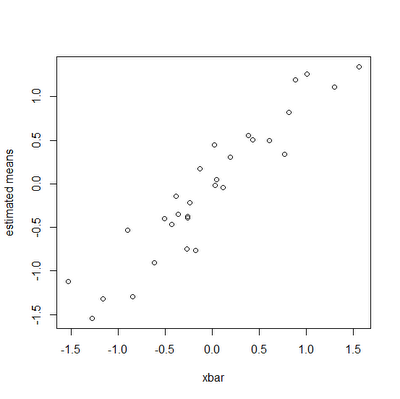## Thursday, December 29, 2011

### New function for comparative analysis with intraspecific data

I recently added a function to phytools called fitBayes for fitting evolutionary models with intraspecific variability. It is in version 0.1-5 (and higher) of the phytools package, and I also just posted the code to my R phylogenetics page. Note that multiple functions are required to run this analysis so it is probably better to just download the phytools package (latest nonstatic version here).

The function takes as input a species phylogeny and a vector of data for one or multiple individuals per species. It then simultaneously samples species means and variances, as well as the parameters of the fitted evolutionary model (presently BM and the λ model). The neat thing about this method is that the tree and evolutionary model can influence the estimated species means (as well as the reverse). In theory (and in simulations) this leads to more accurate estimates of the means for species than when the phylogeny is ignored.

I'm not going to go into great detail about this function - I have a submitted manuscript in which Graham Reynolds (presently a postdoc in my lab) and I describe the approach - however I have posted a little demo, with explanation, below.

First, load phytools and simulate a tree and data for the species means at the tips:

require(phytools)
set.seed(1)
tree<-pbtree(n=30,scale=1)
xbar<-fastBM(tree,sig2=0.5) # true species means

Now, we can generate a vector in which the values are sampled from a normal distribution with means given by xbar:

x<-vector()
for(i in 1:30){
n<-floor(runif(n=1,min=1,max=4))
y<-rnorm(n=n,mean=xbar[i],sd=sqrt(0.2))
names(y)<-rep(names(xbar)[i],length(y))
x<-c(x,y)
}

Now, let's run the MCMC:

X<-fitBayes(tree,x,ngen=100000,model="BM",method="reduced")

Ok, now, let's get our estimates, excluding the first 20,000 generations (201 samples) as burnin:

> results<-colMeans(X[201:1001,])
> results
gen          sig2             a       t1  ...
6.000000e+04  9.427381e-01  5.132597e-02  5.04303  ...

We can, say, plot these estimates against their generating values:

plot(xbar,results[names(xbar)],ylab="estimated means")We can also compare the Bayesian and standard arithmetic means quantitatively. Here, I will compute the sum of squared differences between the estimated means and the underlying generating values:

> SS.bayes<-sum((results[names(xbar)]-xbar)^2)
> SS.bayes
 2.143579
> temp<-aggregate(as.data.frame(x),list(as.factor(names(x))),mean)
> x.arith<-temp[,2]; names(x.arith)<-temp[,1]
> SS.arith<-sum((x.arith[names(xbar)]-xbar)^2)
> SS.arith
 3.953547

Here, at least, taking the tree and evolutionary model into account means we also get much better estimates of the species means. Neat.